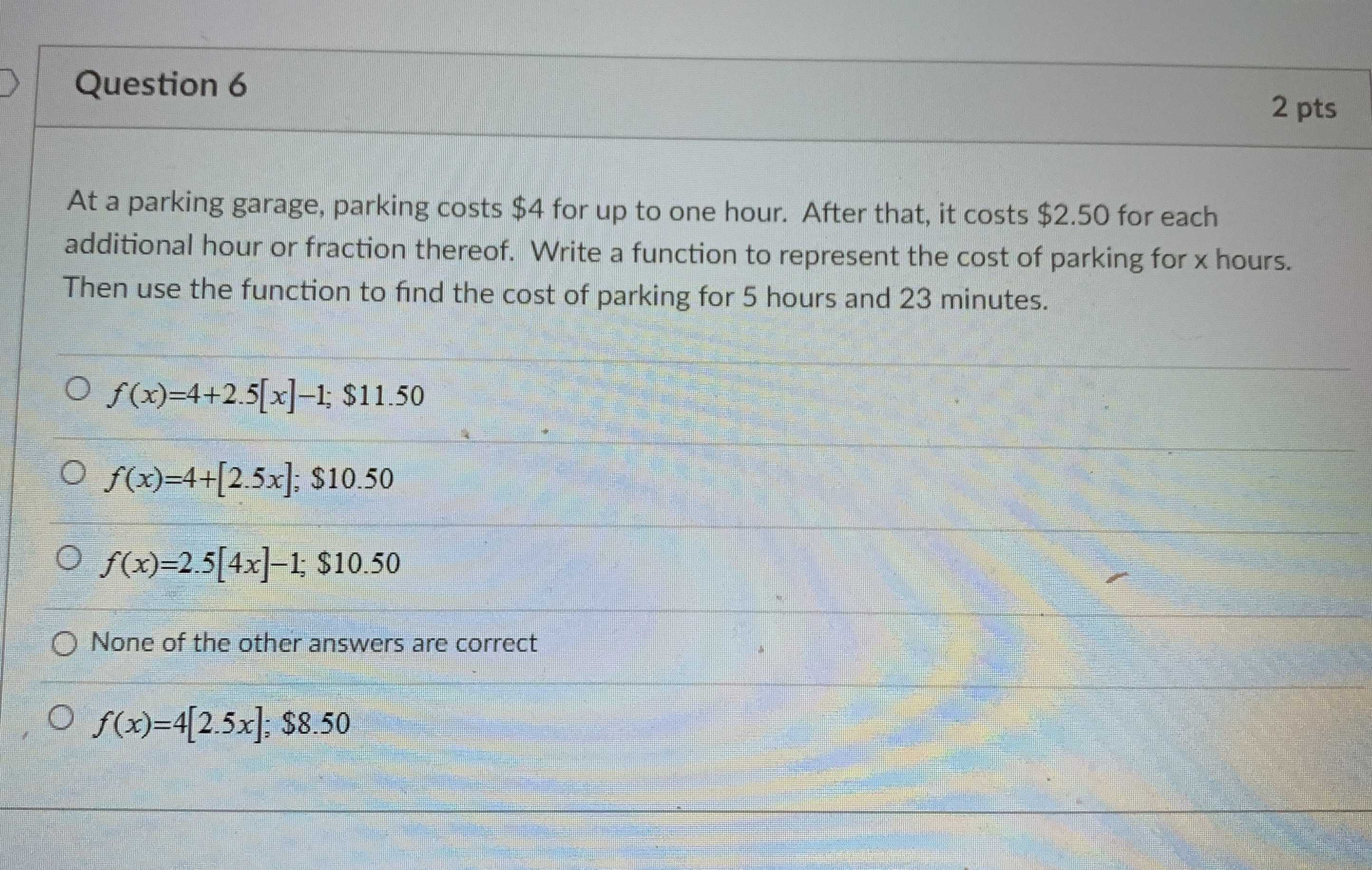### Still have math questions?

Algebra
QuestionAt a parking garage, parking costs $$\ 4$$ for up to one hour. After that, it costs $$\ 2.50$$ for each additional hour or fraction thereof. Write a function to represent the cost of parking for $$x$$ hours. Then use the function to find the cost of parking for $$5$$ hours and $$23$$ minutes.

$$f ( x ) = 4 + 2.5 [ x ] - 1 ; \ 11.50$$

$$f ( x ) = 4 + [ 2.5 x ] ; \ 10.50$$

$$f ( x ) = 2.5 [ 4 x ] - 1 ; \ 10.50$$

None of the other answers are correct

$$f ( x ) = 4 [ 2.5 x ] ; \ 8.50$$